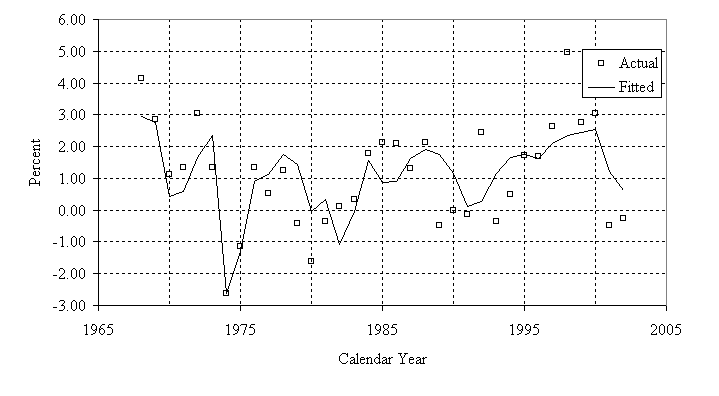## A Stochastic Model of the Long-Range Financial Status of the OASDI Program—September 2004

II. EQUATION SELECTION AND PARAMETER ESTIMATION

### E. REAL AVERAGE COVERED WAGE (PERCENT CHANGE)

The real average covered wage is defined as the ratio of the average nominal OASDI covered wage to the adjusted inflation rate. Because of the expansion of covered employment, the annual growth rate in the real average covered wage differs significantly from the annual growth rate in a real average economy-wide wage series. In the future, however, the annual growth rates in the two measures are expected to be approximately identical since projected coverage changes are insignificant. Hence, the historical variation of the annual percent change in the real average economy-wide wage is used to model the future variation of the annual percent change in the real average covered wage.

The real average economy-wide wage is the ratio of the average nominal wage to the adjusted CPI. The nominal wage is the ratio of wage disbursement as published by the Bureau of Economic Analysis' (BEA) National Income and Product Accounts (NIPA) to civilian employment. Civilian employment is the sum of total wage employment, as published by the BLS from its Household Survey, and total U.S. Armed Forces from the Census Bureau. The BLS periodically introduces improvements to its employment data but does not revise earlier data. However, the BLS has developed adjustment factors to improve the comparability of employment data with earlier years.1 OCACT has used these factors to adjust the wage employment data.

The formula for calculating the annual percent change in the real average wage, given a nominal wage series, is:

Wt = (NWtNWt-1) ⁄ (CPItCPIt-1) −1.

Wt is the annual percent change in the real average wage expressed in decimals in year t; NWt is the level of the nominal average wage in year t; and CPIt is the level of the CPI in year t.

The model estimates the annual percent changes in the real economy-wide wage as a function of the current unemployment rate and the unemployment rate of the previous year, expressed as log-odds ratios, over the period from 1968 to 2002. The value for 1974 was an outlier and therefore was excluded in the development of the equation. The R-squared value was 0.53. The actual and fitted values are shown in figure II.6.

The estimated coefficients and standard error of the regression are then used to simulate the percent change in the real average covered wage. The modified equation is:

Wt = WtTR −0.06ut +0.04ut-1 + εt .                        (9)

In this equation, Wt represents the percent change in the real average covered wage in year t; WtTR represents the percent change in the real average covered wage from the TR04II in year t; ut represents the deviation of the (log-odds transformed) unemployment rate from the TR04II unemployment rate in year t; and εt represents the random error in year t.[D]

### Footnote—

1 For a detailed description of the methodology, refer to the article titled Creating Comparability in CPS Employment Series - www.bls.gov/cps/cpscomp.pdf.